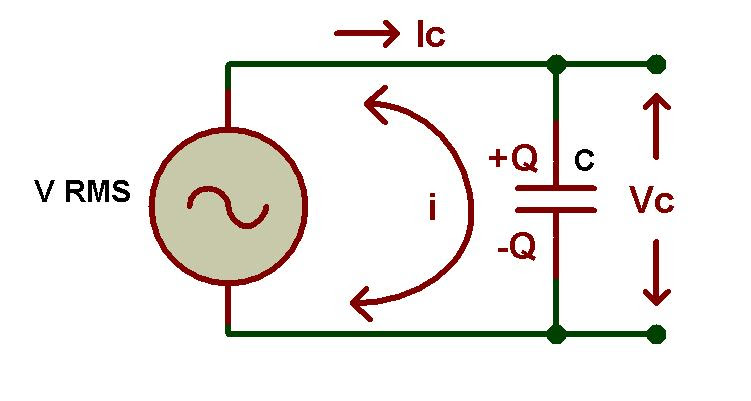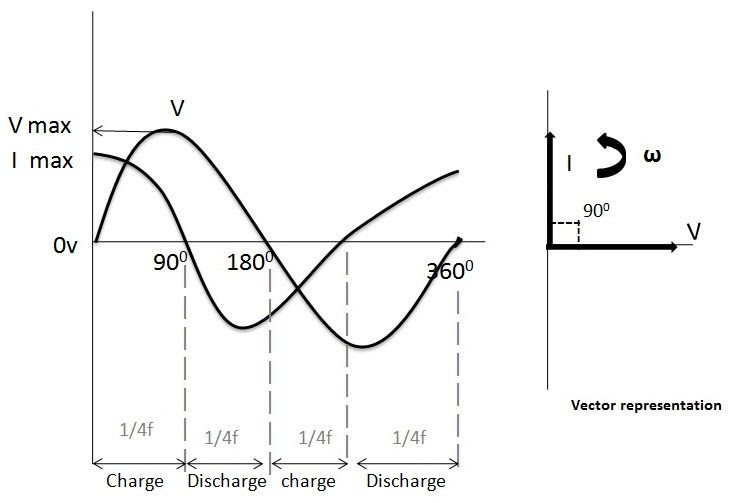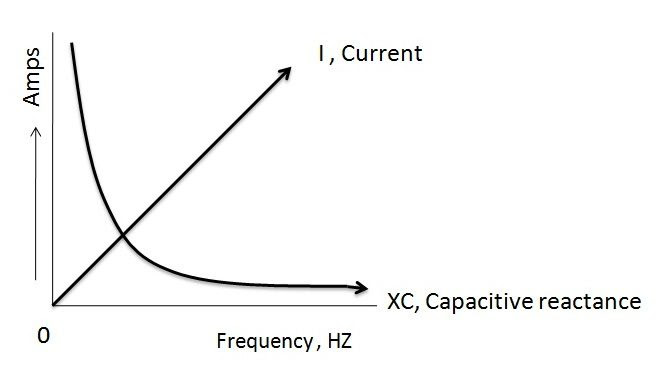# Capacitance in AC Circuits:

Introduction:

When DC supply voltage is applied to the capacitor,the capacitor is charged slowly and finally it reaches to fully charged position. At this point the charging voltage of a capacitor is equal to the supply voltage. Here the capacitor acts as an energy source as long as voltage is applied. Capacitors don’t allow current (i) through them after they fully charged.The current flowing through the circuit depends on the amount of charge in the plates of capacitors and also the current is directly proportional to the rate of change of voltage applied to the circuit. i.e. i = dQ/dt = C dV(t)/dt.
If AC supply voltage is applied to the capacitor circuit then the capacitor charges and discharges continuously depending on the rate of frequency of supply voltage. The capacitance of a capacitor in AC circuits depends on the frequency of supply voltage applied to it. In AC circuits the capacitors allow current when the supply voltage is continuously changing with respect to time.

### AC Capacitor Circuit:In the above circuit we observed that a capacitor is directly connected to the AC supply voltage. Here the capacitor continuously charges and discharges depending on the changes in supply voltage, because the AC supply voltage value is constantly increases and decreases. We all know that the current flowing through the circuit is directly proportional to the rate of change of voltage applied.
Here the charging current has its high value, if the supply voltage crosses its value from positive half cycle to the negative half cycle and vice versa. i.e. at  00 and 1800 in sine wave signal. The current through the capacitor has its minimum value when the supply voltage in sine wave crosses over at its maximum or minimum peak value (Vm). Hence we can say that the charging current flowing through the circuit is maximum or minimum depending on the supply voltage levels in sine wave.

### AC Capacitor Phasor Diagram:The phasor diagram of AC capacitor is shown in the above figure, here the voltage and currents are represented in sine wave forms. In the above figure we observed that at 0the charging current is at its maximum value because the voltage is increasing slowly in positive direction. At 900 there is no current flow through the capacitor because at this point the supply voltage is at its maximum peak value.
At 1800 point the voltage slowly decreases to zero and current is at maximum value in the negative direction. Again the charging reaches to its maximum value at 3600, because at this point the supply voltage is at minimum value.
From the waveforms in the above figure we can see that, the current is leading the voltage by 900. Hence we can say that in an ideal capacitor circuit the AC voltage lags the current by 900.

### Capacitive Reactance:

We know that the current flowing through the capacitor is directly proportional the rate of change of applied voltage but capacitors also offer some form of resistance against the current flow same as like resistors. This resistance of capacitors in AC circuits is called as capacitive reactance or commonly known as reactance. Capacitive reactance is the property of a capacitor which opposes the flow of current in AC circuits. It is represented with symbol Xc and measured in Ohms same as like resistance.
We need some extra energy over capacitive reactance to charge up a capacitor in the circuit. This value is inversely proportional to the capacitance value and the frequency of supply voltage.
Xc∝ 1/c and Xc∝ 1/f.
The equation for capacitive reactance and parameters which influences them are discussed in below.
Capacitive Reactance,
XC = 1/2πfC = 1/ωC
Here,
XC = Reactance of capacitor
f = frequency in HZ
C = Capacitance of a capacitor in Farads
ω (omega) = 2πf
From the above equation we understood that capacitive reactance is high where the frequency and capacitance values are at low and at this stage the capacitor acts as a perfect resistor. If the frequency of supply voltage is high then the reactance value of capacitor is low and also at this stage capacitor acts as a good conductor. From the above equation it is clear that the reactance is zero if the frequency is infinity and the reactance value is infinity where the frequency is at zero.

### Capacitive Reactance against Frequency:The above figure shows the relation between the capacitive reactance, current and frequency of the supply voltage. Here we observed that if frequency is low then the reactance is high. The charging current increases with increase in frequency, because the rate of change of voltage increases with time.The reactance is at infinite value where the frequency is zero and vice versa.

### AC Capacitance - Example No1:

Find the rms value of current flowing through the circuit having 3uF capacitor connected to 660V and 40Hz supply.
Capacitive Reactance,
XC = 1/2πfC
Here,
f = 40HZ
C = 3uF
Vrms = 660V
Now,
XC = 1/(2 × 3.14 × 40HZ  × 3 × 10-6) = 1326Ω
Irms = Vrms/XC = 660V/1326Ω = 497mA

### AC Capacitance - Example No2:

Find the rms value of current flowing through the circuit having 5uF capacitor connected to 880V and 50Hz supply.
Capacitive Reactance,
XC = 1/2πfC
Here,
f = 50HZ
C = 5uF
Vrms = 880V
Now,
XC = 1/(2 × 3.14 × 50HZ × 5 × 10-6) = 636Ω
Irms = Vrms/XC = 880V/636Ω = 1.38 A
From the above two examples practically we observed that the reactance of a capacitor depends on the frequency of the supply voltage and it is inversely proportional relation. In example 1 , the reactance is 1326Ω for the frequency of 40HZ but the reactance value decreases to 636Ω when the frequency increases to 50HZ which is shown in example 2.Hence it is clear that the reactance of a capacitor is inversely proportional to the frequency and capacitance.
REFERENCE // Electronics Hub